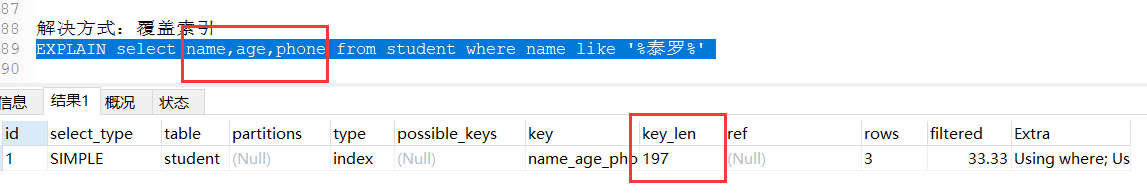# MySQL常見優化方案匯總

mysql優化是我們日常工作經常遇到的問題，今天給大傢說下MySQL常見的幾種優化方案。

## 思考sql優化的幾個地方，我把他做瞭個分類，方便理解

select [字段 優化1]：主要是覆蓋索引
from []
where [條件 優化2]
union [聯合查詢 優化3]

```CREATE TABLE `student` (
`id` int(11) NOT NULL AUTO_INCREMENT COMMENT '主鍵',
`name` varchar(50) DEFAULT NULL COMMENT '姓名',
`age` int(11) DEFAULT NULL COMMENT '年齡',
`phone` varchar(12) DEFAULT NULL,
`create_time` datetime DEFAULT NULL COMMENT '創建時間',
PRIMARY KEY (`id`)
) ENGINE=InnoDB DEFAULT CHARSET=utf8;```

key_len：根據這個值，就可以判斷索引使用情況，特別是在組合索引的時候，判斷所有的索引字段是否都被查詢用到。

### key_len計算方式簡單介紹

latin1占用1個字節，gbk占用2個字節，utf8占用3個字節

varchar(10)：10*3

char(10)：10*3+2

int：4

varchar(10)：10*3+1

char(10)：10*3+2+1

int：4+1

`alter table studen add index name_age_phone(name, age, phone);`

```insert into student(name,age,phone,create_time) values('賽文',1000,'15717177664',now());
insert into student(name,age,phone,create_time) values('雷歐',1200,'15733337664',now());
insert into student(name,age,phone,create_time) values('泰羅',800,'15714447664',now());```

## 一、優化點1：字段優化

### 覆蓋索引盡量用

```#未覆蓋索引
EXPLAIN SELECT * FROM student WHERE NAME = '泰羅' and age =1000 and phone='15717177664';
#覆蓋瞭索引
EXPLAIN SELECT name,age,phone FROM student WHERE NAME = '泰羅' and age =1000 and phone='15717177664';
#包含瞭索引
EXPLAIN SELECT name FROM student WHERE NAME = '泰羅' and age =1000 and phone='15717177664';
#加上主鍵也還是覆蓋索引
EXPLAIN SELECT id, name,age,phone FROM student WHERE NAME = '泰羅' and age =1000 and phone='15717177664';```## 二、優化點2：where優化

### 1.盡量全值匹配

```EXPLAIN SELECT * FROM student WHERE NAME = '賽文';
EXPLAIN SELECT * FROM student WHERE NAME = '雷歐' AND age = 1200;
EXPLAIN SELECT * FROM student WHERE NAME = '泰羅' AND age = 800 AND phone = '15714447664';```### 2.最佳左前綴法則### 3.范圍條件放最後

`EXPLAIN SELECT * FROM student WHERE NAME = '泰羅' AND age = 1000 AND phone = '15717177664';``EXPLAIN SELECT * FROM student WHERE NAME = '泰羅' AND age > 800 AND phone = '15717177664';`

key_len=name(153)+age(5)### 4.不在索引列上做任何操作

```EXPLAIN SELECT * FROM student WHERE NAME = '泰羅';
EXPLAIN SELECT * FROM student WHERE left(NAME,1) = '泰羅';```### 5.不等於要甚用

mysql 在使用不等於 (!= 或者 <>) 的時候無法使用索引會導致全表掃描

```#有使用到索引
EXPLAIN SELECT * FROM student WHERE NAME = '泰羅';
#不等於查詢，未使用到索引
EXPLAIN SELECT * FROM student WHERE NAME != '泰羅';
EXPLAIN SELECT * FROM student WHERE NAME <> '泰羅';

#如果定要需要使用不等於,請用覆蓋索引
EXPLAIN SELECT name,age,phone FROM student WHERE NAME != '泰羅';
EXPLAIN SELECT name,age,phone FROM student WHERE NAME <> '泰羅';```### 6.Null/Not null有影響```EXPLAIN select * from student where name is null;
EXPLAIN select * from student where name is not null;```### 7、Like 查詢要當心 like

```#like 以通配符開頭('%abc...')mysql 索引失效會變成全表掃描的操作
#索引有效
EXPLAIN select * from student where name ='泰羅';
#索引失效
EXPLAIN select * from student where name like '%泰羅%';
#索引失效
EXPLAIN select * from student where name like '%泰羅';
#索引有效
EXPLAIN select * from student where name like '泰羅%';

EXPLAIN select name,age,phone from student where name like '%泰羅%';```### 8.字符類型加引號## 三、優化3

### 1.OR 改 UNION 效率高

```未使用索引
EXPLAIN select * from student where name='泰羅' or name = '雷歐';

EXPLAIN
select * from student where name='泰羅'
UNION
select * from student where name = '雷歐';

EXPLAIN select name,age from student where name='泰羅' or name = '雷歐';```